NCERT Exemplar - MCQs

Chapter 5 Class 12 Continuity and Differentiability
Serial order wise

## (D) None of these

This question is similar to Ex 5.1, 32 - Chapter 5 Class 12 and Ex 5.2, 9 - Chapter 5 Class 12 Continuity and Differentiability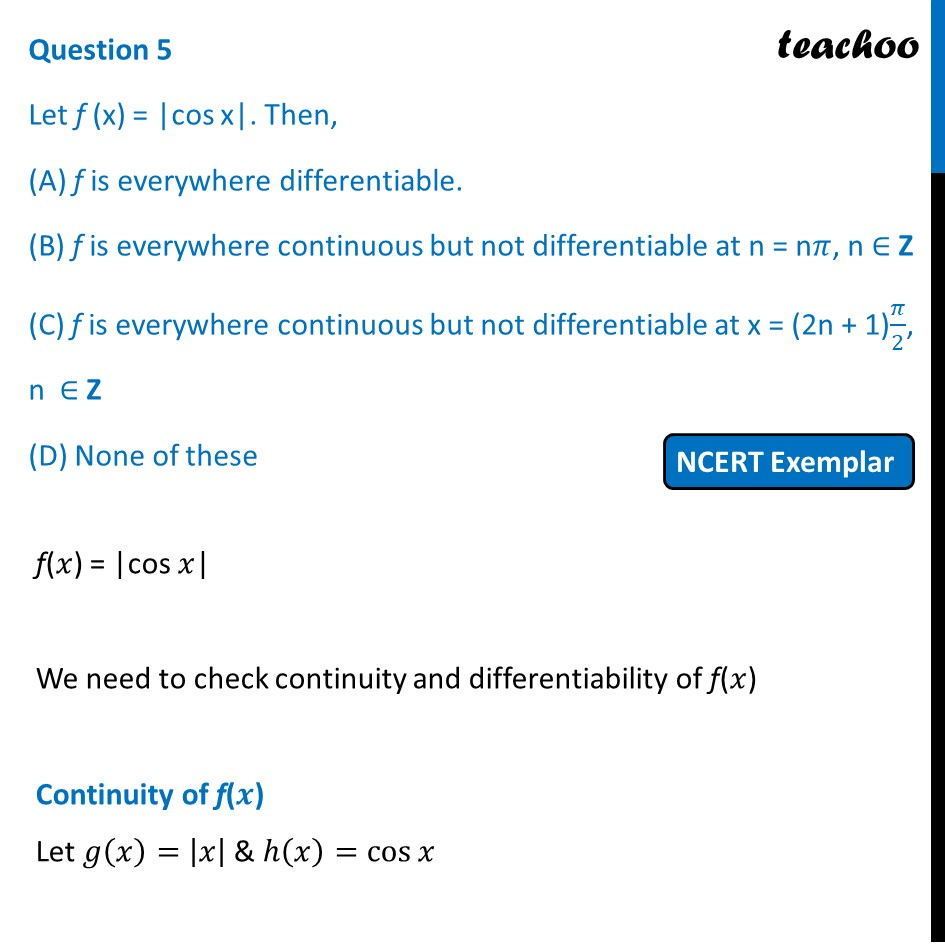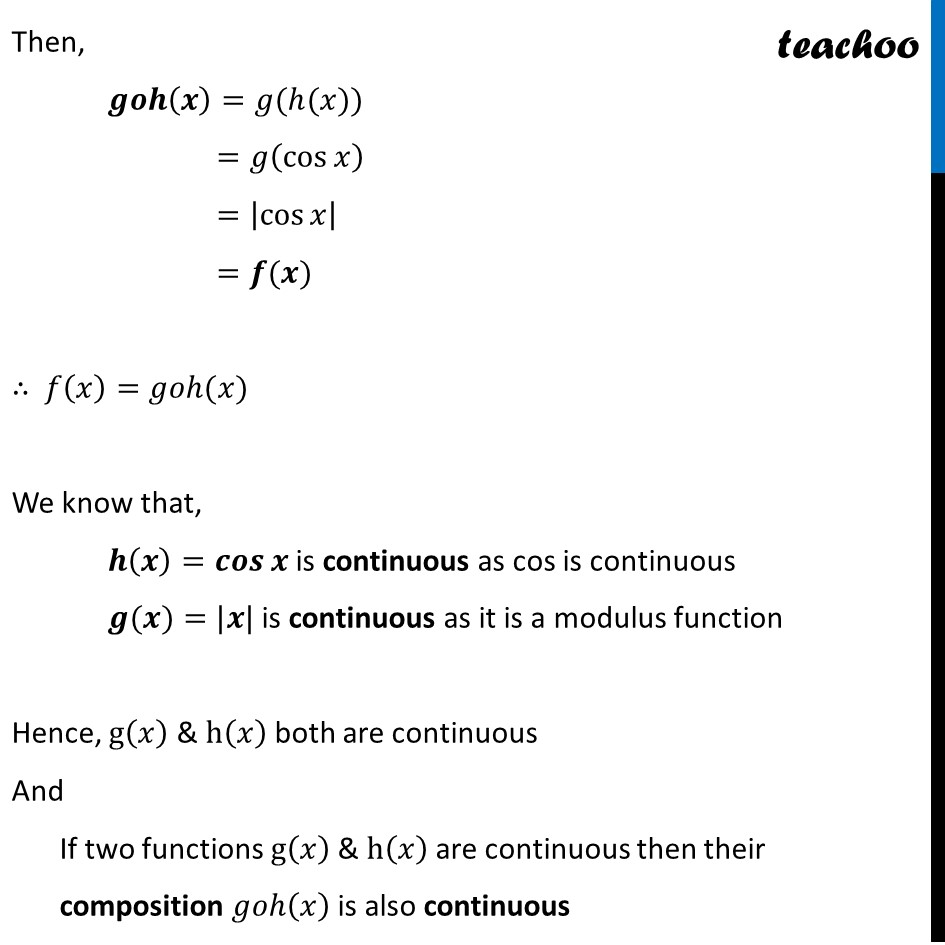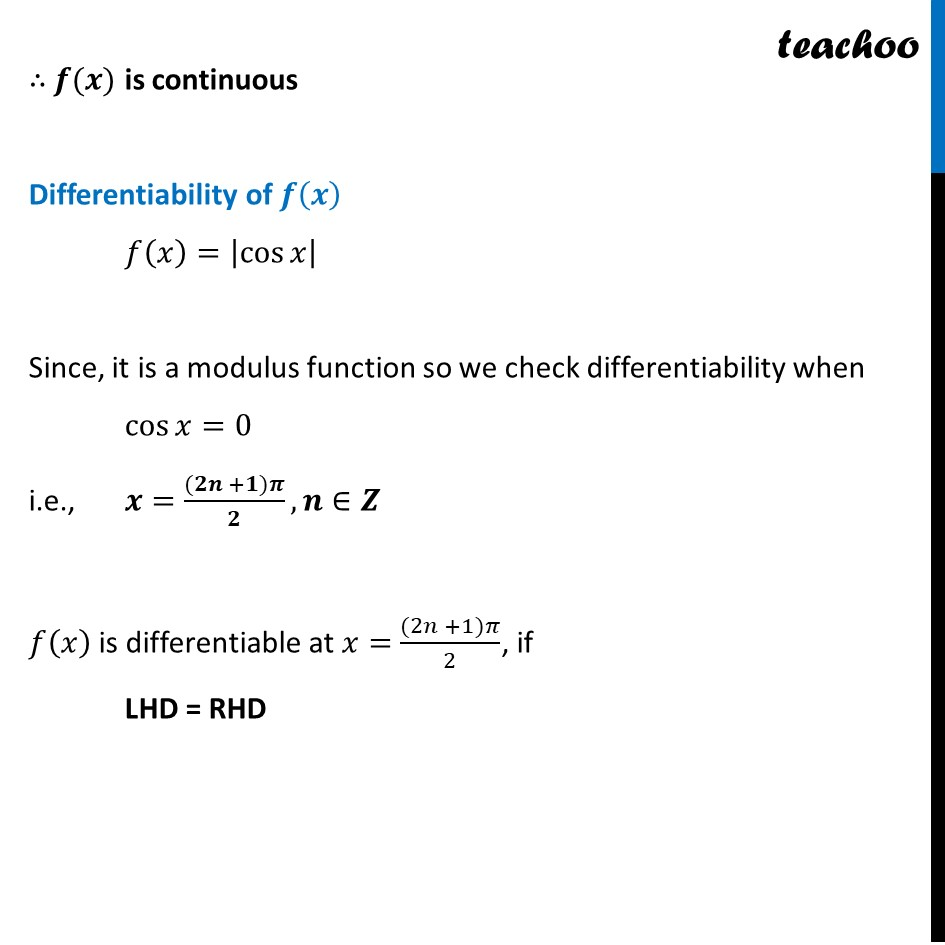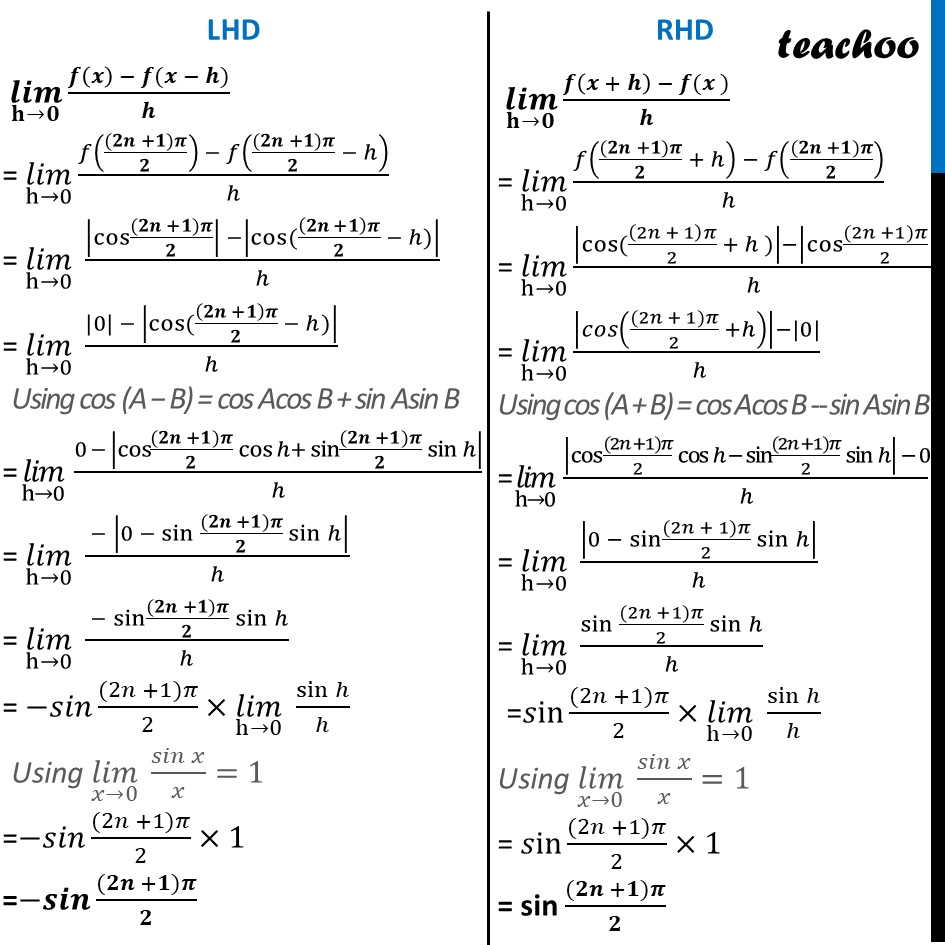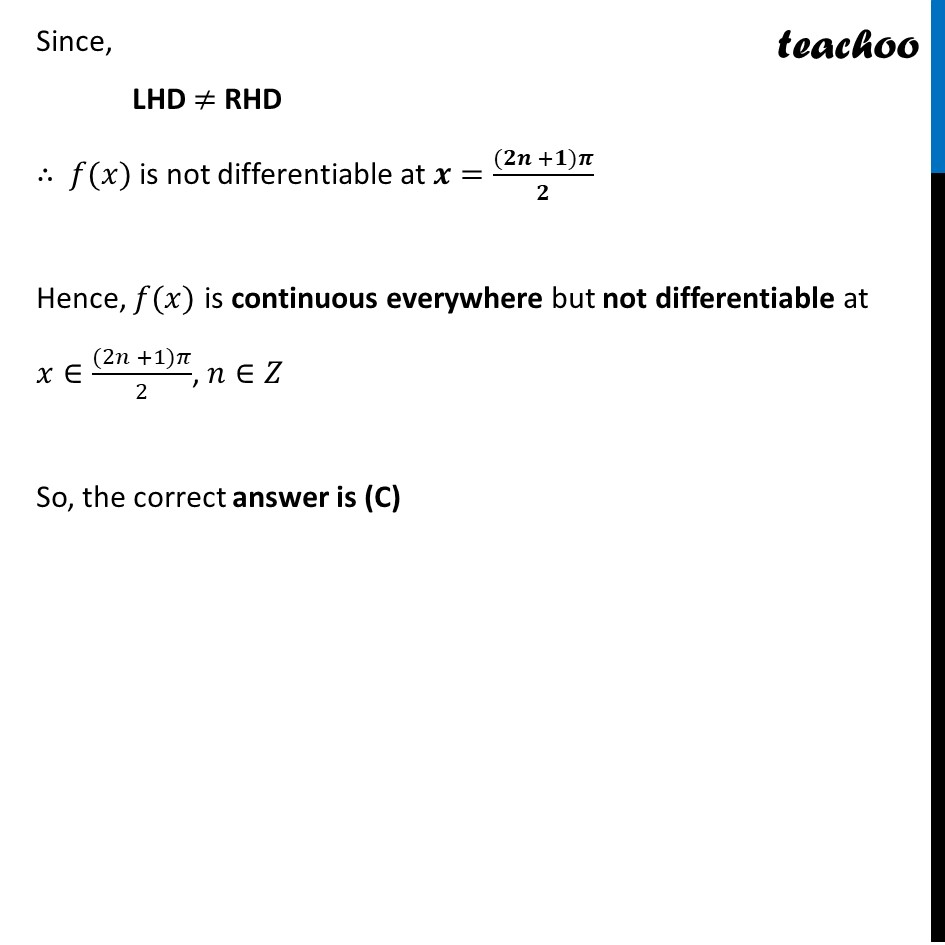Learn in your speed, with individual attention - Teachoo Maths 1-on-1 Class

### Transcript

Question 5 Let f (x) = |cos x|. Then, (A) f is everywhere differentiable. (B) f is everywhere continuous but not differentiable at n = n𝜋, n ∈ Z (C) f is everywhere continuous but not differentiable at x = (2n + 1)𝜋/2, n ∈ Z (D) None of these f(𝑥) = |cos 𝑥| We need to check continuity and differentiability of f(𝑥) Continuity of f(𝒙) Let 𝑔(𝑥)=|𝑥| & ℎ(𝑥)=cos⁡𝑥 Then, 𝒈𝒐𝒉(𝒙)=𝑔(ℎ(𝑥)) =𝑔(cos⁡𝑥 ) =|cos⁡𝑥 | =𝒇(𝒙) ∴ 𝑓(𝑥)=𝑔𝑜ℎ(𝑥) We know that, 𝒉(𝒙)=𝒄𝒐𝒔⁡𝒙 is continuous as cos is continuous 𝒈(𝒙)=|𝒙| is continuous as it is a modulus function Hence, g(𝑥) & h(𝑥) both are continuous And If two functions g(𝑥) & h(𝑥) are continuous then their composition 𝑔𝑜ℎ(𝑥) is also continuous ∴ 𝒇(𝒙) is continuous Differentiability of 𝒇(𝒙) 𝑓(𝑥)=|cos⁡𝑥 | Since, it is a modulus function so we check differentiability when cos⁡𝑥=0 i.e., 𝒙=((𝟐𝒏 +𝟏)𝝅)/𝟐, 𝒏∈𝒁 𝑓(𝑥) is differentiable at 𝑥=((2𝑛 +1)𝜋)/2, if LHD = RHD (𝒍𝒊𝒎)┬(𝐡→𝟎) (𝒇(𝒙) − 𝒇(𝒙 − 𝒉))/𝒉 = (𝑙𝑖𝑚)┬(h→0) (𝑓(((𝟐𝒏 +𝟏)𝝅)/𝟐) − 𝑓(((𝟐𝒏 +𝟏)𝝅)/𝟐 − ℎ))/ℎ = (𝑙𝑖𝑚)┬(h→0) (|cos⁡〖((𝟐𝒏 +𝟏)𝝅)/𝟐〗 | −|cos⁡〖((𝟐𝒏 +𝟏)𝝅/𝟐 − ℎ)〗 |)/ℎ = (𝑙𝑖𝑚)┬(h→0) (|0| − |cos⁡〖((𝟐𝒏 +𝟏)𝝅/𝟐 − ℎ)〗 |)/ℎ Using cos (A − B) = cos Acos B + sin Asin B = (𝑙𝑖𝑚)┬(h→0) (0 − |cos⁡〖((𝟐𝒏 +𝟏)𝝅)/𝟐 cos⁡〖ℎ+ sin⁡〖((𝟐𝒏 +𝟏)𝝅)/𝟐 sin⁡ℎ 〗 〗 〗 |)/ℎ = (𝑙𝑖𝑚)┬(h→0) ( − |0 − 〖sin 〗⁡〖((𝟐𝒏 +𝟏)𝝅)/𝟐 sin⁡ℎ 〗 |)/ℎ = (𝑙𝑖𝑚)┬(h→0) ( −sin⁡〖((𝟐𝒏 +𝟏)𝝅)/𝟐 sin⁡ℎ 〗)/ℎ = −𝑠𝑖𝑛 ((2𝑛 +1)𝜋)/2×(𝑙𝑖𝑚)┬(h→0) sin⁡ℎ/ℎ Using (𝑙𝑖𝑚)┬(𝑥→0) 𝑠𝑖𝑛⁡𝑥/𝑥=1 =−𝑠𝑖𝑛 ((2𝑛 +1)𝜋)/2×1 =−𝒔𝒊𝒏 ((𝟐𝒏 +𝟏)𝝅)/𝟐 (𝒍𝒊𝒎)┬(𝐡→𝟎) (𝒇(𝒙 + 𝒉) − 𝒇(𝒙 ))/𝒉 = (𝑙𝑖𝑚)┬(h→0) (𝑓(((𝟐𝒏 +𝟏)𝝅)/𝟐 + ℎ) − 𝑓(((𝟐𝒏 +𝟏)𝝅)/𝟐))/ℎ = (𝑙𝑖𝑚)┬(h→0) (|cos⁡〖((2𝑛 + 1)𝜋/2 + ℎ )〗 |−|cos⁡〖((2𝑛 +1)𝜋)/2〗 |)/ℎ = (𝑙𝑖𝑚)┬(h→0) (|𝑐𝑜𝑠⁡(((2𝑛 + 1)𝜋)/2 +ℎ) |−|0|)/( ℎ) Using cos (A + B) = cos Acos B -- sin Asin B = (𝑙𝑖𝑚)┬(h→0) (|〖cos 〗⁡〖((2𝑛 +1)𝜋)/2 cos⁡〖ℎ − sin⁡〖((2𝑛 +1)𝜋)/2 sin⁡ℎ 〗 〗 〗 | − 0)/ℎ = (𝑙𝑖𝑚)┬(h→0) |0 − sin⁡〖((2𝑛 + 1)𝜋)/2 sin⁡ℎ 〗 |/ℎ = (𝑙𝑖𝑚)┬(h→0) 〖sin 〗⁡〖((2𝑛 +1)𝜋)/2 sin⁡ℎ 〗/ℎ =𝑠in ((2𝑛 +1)𝜋)/2×(𝑙𝑖𝑚)┬(h→0) sin⁡ℎ/ℎ Using (𝑙𝑖𝑚)┬(𝑥→0) 𝑠𝑖𝑛⁡𝑥/𝑥=1 = 𝑠in ((2𝑛 +1)𝜋)/2×1 = sin ((𝟐𝒏 +𝟏)𝝅)/𝟐 Since, LHD ≠ RHD ∴ 𝑓(𝑥) is not differentiable at 𝒙=((𝟐𝒏 +𝟏)𝝅)/𝟐 Hence, 𝑓(𝑥) is continuous everywhere but not differentiable at 𝑥∈((2𝑛 +1)𝜋)/2, 𝑛∈𝑍 So, the correct answer is (C)